# M,R a = 0 14. A body consisted of an uniform rod of mass m and length L, and a disk of mass M and radius R can rotate a...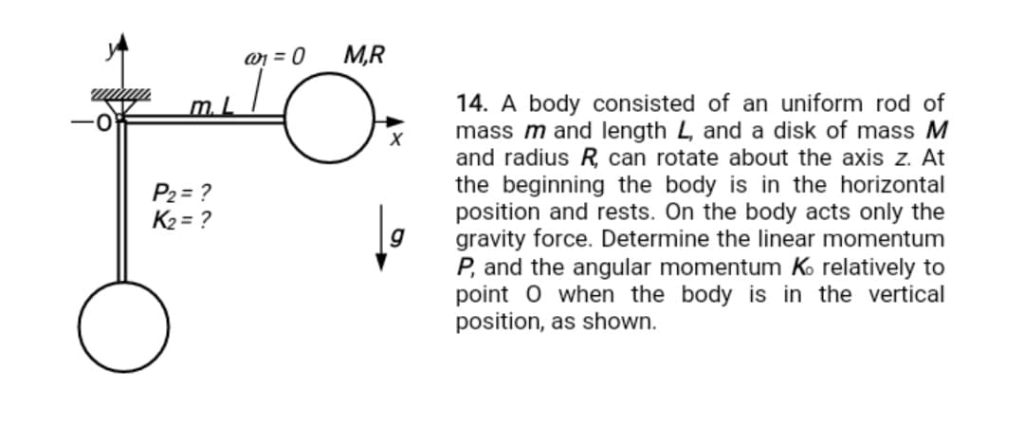M,R a = 0 14. A body consisted of an uniform rod of mass m and length L, and a disk of mass M and radius R can rotate about the axis z. At the beginning the body is in the horizontal position and rests. On the body acts only the gravity force. Determine the linear momentum P, and the angular momentum K relatively to point O when the body is in the vertical position, as shown. m. X P2=? K2=?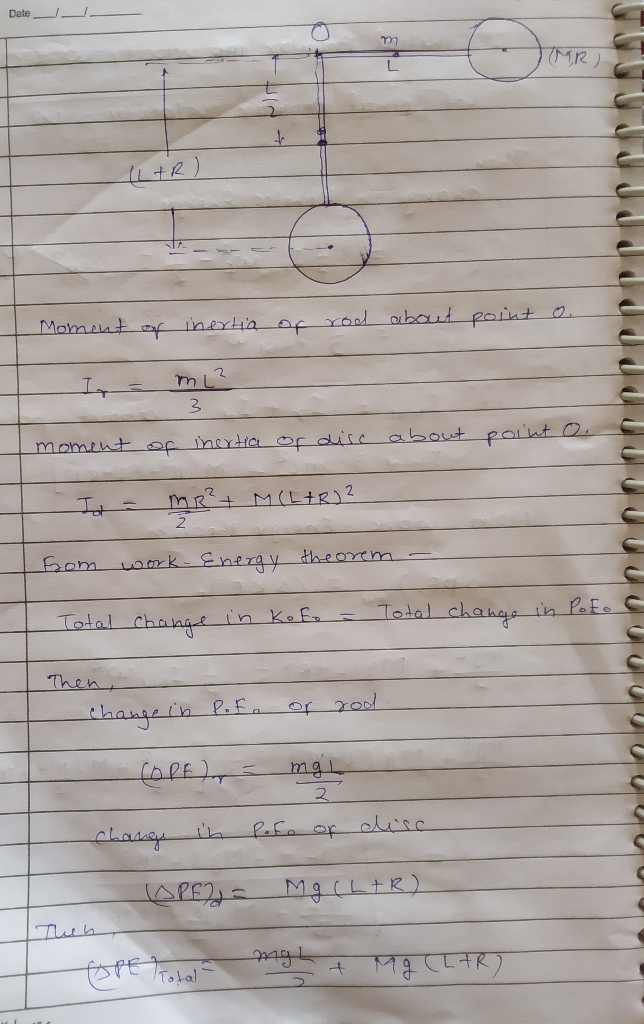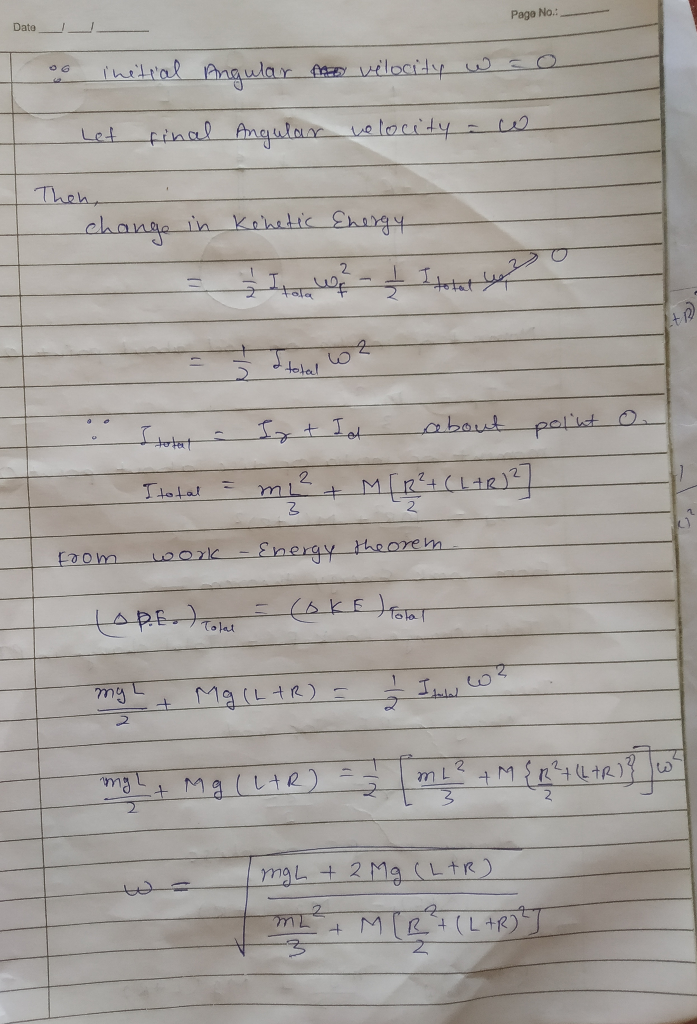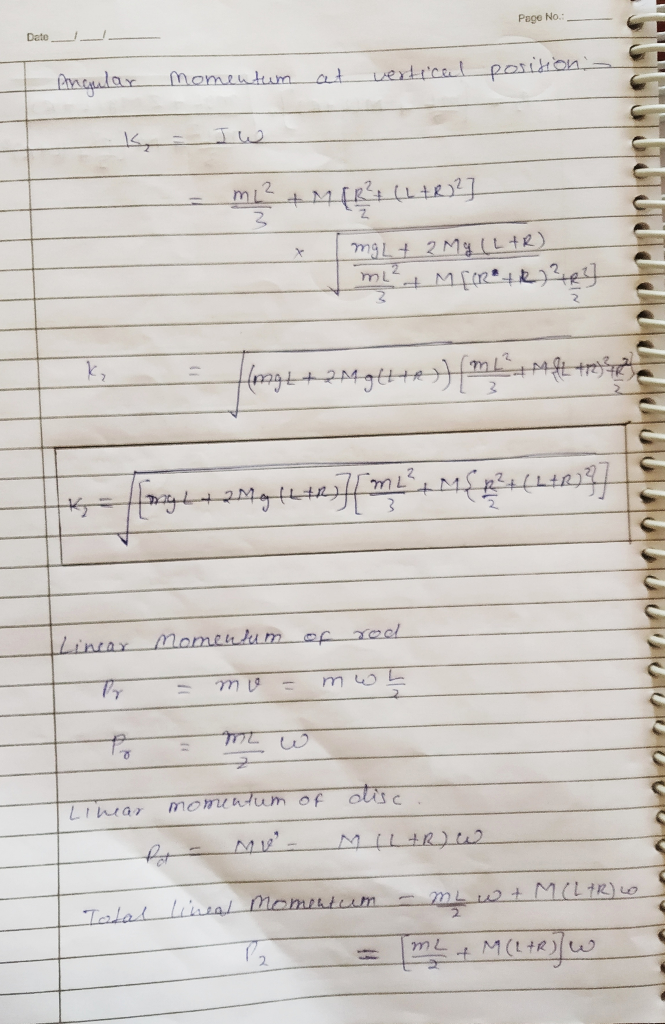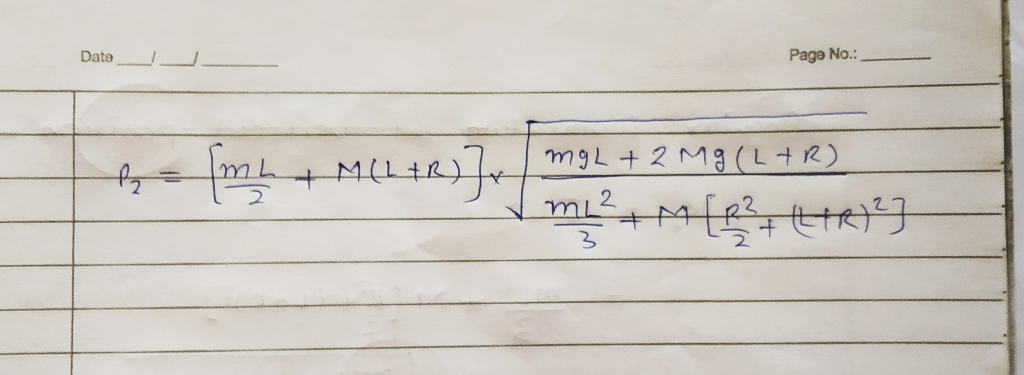##### Add Answer of: M,R a = 0 14. A body consisted of an uniform rod of mass m and length L, and a disk of mass M and radius R can rotate a...
More Homework Help Questions Additional questions in this topic.